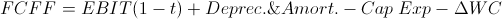# Free Cash Flow to Firm (FCFF)

FCFF Calculator (Click Here or Scroll Down)The free cash flow to firm formula is capital expenditures and change in working capital subtracted from the product of earnings before interest and taxes (EBIT) and one minus the tax rate(1-t).

The free cash flow to firm formula is used to calculate the amount available to debt and equity holders.

## Variables of the FCFF Formula

Earnings before interest and taxes, EBIT, is, as it suggests, the earnings from a company's operations before adjusting for interest expense and taxes. EBIT can be found on the company's income statement or calculated from the cash flow statement. The free cash flow to firm formula does adjust for taxes by multiplying EBIT by one minus the tax rate.

Capital expenditures (Capex) is the capital used to fund operations in the long run. Capital expenditures can be found on a company's cash flow statement.

Working capital is capital used to fund operations in the short run. Working capital is current assets minus current liabilities. As opposed to longer term capital expenditures, working capital connotes expenses due within one year or less. The change in working capital can be calculated using a company's balance sheet.

## FCFF Formula vs. FCFE Formula

Free cash flow to firm differs from free cash flow to equity in that it calculates the amount available to both debt and equity holders, as opposed to simply equity holders. One part of how this difference is shown in the free cash flow to firm formula is by instead of using net income, EBIT adjusted for taxes is used. This allows interest expenses to be included as they are paid to debt holders.

Another difference between FCFF and FCFE is that the free cash flow to firm formula does not subtract out change in debt. As with other differences listed above, this difference is applied in order to include the amount available to debt.

## Use of the FCFF Formula

As stated in the prior section, the free cash flow to firm formula is used to determine how much debt and equity holders have available. Apart from this general use of the free cash flow to firm, it may also used in valuation models for a company's stock using the FCFF approach to discounting future cash flows. In this approach, FCFF is used in place of dividends.

New to Finance?